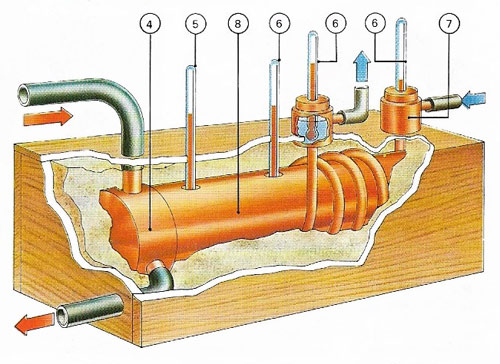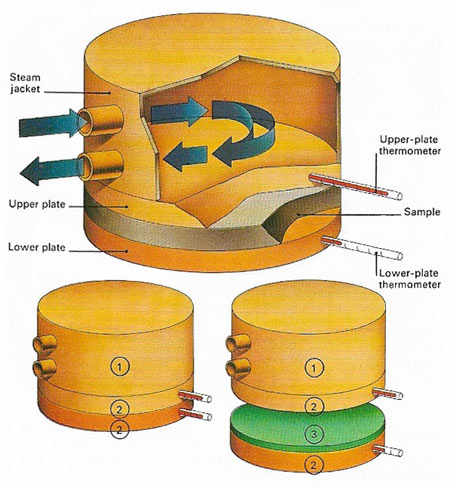A# conductivityFig 1. Thermal conductivity is the amount of heat passing in unit time across unit cross-section per unit of temperature gradient. It can be measured by noting the time taken for a known quantity of heat to pass through a sample. To plates of equal area (2) are put against the material 93). Thermometers on each side measure the temperatures as the upper plate is heated by a steam jacket (1).Fig 2. Materials of high conductivity are tested in a cylinder (8) heated at one end by steam (4) to 100°C (5). Other thermometers (6) measure the temperature of the sample and the rise in temperature of water circulating through a jacket attached to the other end (7), a give a way of calculating conductivity.

## Electrical conductivity

Electrical conductivity, or specific conductance, is the conductance of a 1-meter cube of a substance, measured between opposite faces. Having units of siemens per meter, conductivity is the reciprocal of resistivity, and expresses the substance's ability to conduct electricity.

The equivalent conductivity, Λ, of an electrolytic solution is the conductivity of a solution divided by its concentration in gram-equivalents (see equivalent weight) per cubic meter, and is usually measured with an electrolytic cell in a Wheatstone bridge. The degree of ionic dissociation (α) was found by Arrhenius to be give by α = Λ/Λ0, where Λ0 is the value of Λ extrapolated to zero concentration; this equation has since been shown to apply only to weak electrolytes.

## Thermal conductivity

Thermal conductivity is a measure of the ability of a substance to conduct heat (see thermal conduction). For a block of material of cross section A, the energy transferred per unit time E/t, between faces a distance, l, apart is given by E/t = λA(T2 - T1)/l, where λ is the conductivity and T2 and T1 are the temperatures of the faces. This assumes that the opposite faces are parallel and that there is no heat loss through the sides of the block. The SI unit of thermal conductivity is therefore J s-1 m-1 K-1.